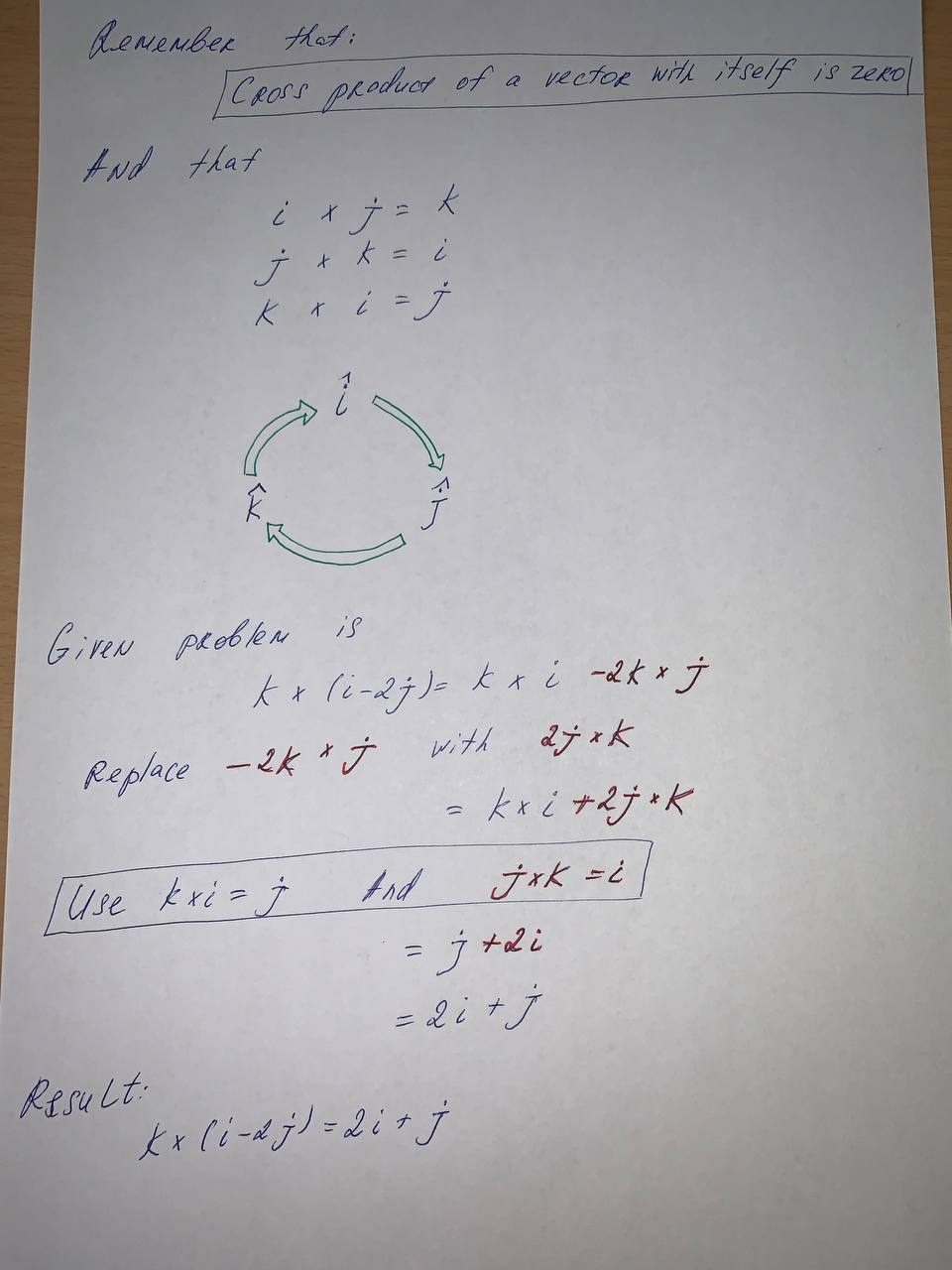# Find the maximum rate of change off at the given point and the direction in which it occurs. f(x, y) = 4y \sqrt{x}, (4,1)sagnuhh 2021-05-28 Answered
Find the maximum rate of change off at the given point and the direction in which it occurs.
$f\left(x,y\right)=4y\sqrt{x},\left(4,1\right)$
You can still ask an expert for help

• Questions are typically answered in as fast as 30 minutes

Solve your problem for the price of one coffee

• Math expert for every subject
• Pay only if we can solve itpivonie8................